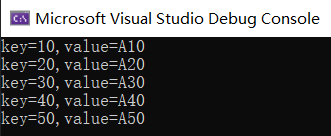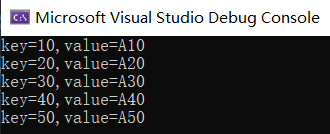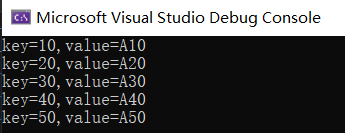# 遍历 Dictionary，你会几种方式？

## 二： 使用 foreach 遍历


var dict = new Dictionary<int, string>()
{
 = "A10",
 = "A20",
 = "A30",
 = "A40",
 = "A50"
};



### 1. 直接 foreach dict


foreach (var item in dict)
{
Console.WriteLine($"key={item.Key},value={item.Value}"); }这里的 item 是底层在 MoveNext 的过程中用 KeyValuePair 包装出来的，如果你不信的话，看下源码呗：  public bool MoveNext() { while ((uint)_index < (uint)_dictionary._count) { ref Entry reference = ref _dictionary._entries[_index++]; if (reference.next >= -1) { _current = new KeyValuePair<TKey, TValue>(reference.key, reference.value); return true; } } }  ### 2. foreach 中 使用 KeyPairValue 解构 刚才你也看到了 item 是 KeyValuePair 类型，不过🐂👃的是 netcore 对 KeyValuePair 进行了增强，增加了 Deconstruct 函数用来解构 KeyValuePair，代码如下：  public readonly struct KeyValuePair<TKey, TValue> { private readonly TKey key; private readonly TValue value; public TKey Key => key; public TValue Value => value; public KeyValuePair(TKey key, TValue value) { this.key = key; this.value = value; } public void Deconstruct(out TKey key, out TValue value) { key = Key; value = Value; } }  有了这个解构函数，你就可以在遍历的过程中直接拿到 key，value，而不是包装的 KeyValuePair，这在 netframework 中可是不行的哈，实现代码如下：  foreach ((int key, string value) in dict) { Console.WriteLine($"key={key},value={value}");
}### 3. foreach keys


foreach (var key in dict.Keys)
{
Console.WriteLine($"key={key},value={dict[key]}"); }说到这里，不知道你是否有一个潜意识，那就是 dict 只能通过 foreach 进行遍历，真相是不是这样的呢？ 要寻找答案，还是回头看一下 foreach 是如何进行遍历的。  public struct Enumerator : IEnumerator<KeyValuePair<TKey, TValue>>, IDisposable, IEnumerator, IDictionaryEnumerator { public bool MoveNext() { while ((uint)_index < (uint)_dictionary._count) { ref Entry reference = ref _dictionary._entries[_index++]; if (reference.next >= -1) { _current = new KeyValuePair<TKey, TValue>(reference.key, reference.value); return true; } } _index = _dictionary._count + 1; _current = default(KeyValuePair<TKey, TValue>); return false; } }  仔细看这个 while 循环，你就应该明白，本质上它也是对 entries 数组进行遍历，那底层都用了 while，我是不是可以用 for 来替换然后循环 dict 呢？哈哈，反正就是模仿呗。 ## 三：使用 for 遍历 为了把 MoveNext 中的代码模拟出来，重点在于这条语句： ref Entry reference = ref _dictionary._entries[_index++];, 其实很简单，_entries 数组内容的提取可以用 Linq 的 ElementAt 方法，是不是~~~ ，改造后的代码如下：  for (int i = 0; i < dict.Count; i++) { (int key, string value) = dict.ElementAt(i); Console.WriteLine($"key={key},value={dict[key]}");
}public static TSource ElementAt<TSource>(this IEnumerable<TSource> source, int index)
{
IList<TSource> list = source as IList<TSource>;
if (list != null)
{
return list[index];
}
if (index >= 0)
{
using (IEnumerator<TSource> enumerator = source.GetEnumerator())
{
while (enumerator.MoveNext())
{
if (index == 0)
{
return enumerator.Current;
}
index--;
}
}
}
}



## 四：总结posted @ 2020-09-28 09:35  一线码农  阅读(960)  评论(6编辑  收藏